# Basic Relationships, Concepts and Laws of Electric Circuits

March 22, 2020 by Robert Keim
This video tutorial explains fundamental laws and relationships that help us to understand, analyze, and design electrical systems and electronic devices.

The primary task of hardware-focused electrical engineers is circuit design. Notice how we repeatedly use this word “circuit” when referring to an interconnected group of sources, resistors, capacitors, and so forth. “Circuit” is not only an engineering word; it is a normal English word that refers to (among other things) some sort of route or movement that starts and ends at the same place.

Electronic design is fundamentally about electric circuits, that is, networks that allow electric charge to start at a source, move through a continuous pathway of conductors and components, and return to the source. This video tutorial will provide an overview of important scientific laws and mathematical relationships that describe the behavior of voltages and currents in the context of an electric circuit. If you want to explore these topics in greater detail, please refer to the AAC textbook.

## Electron Flow vs. Current Flow

In the world of electrical engineering, current flows from higher voltage to lower voltage. For example: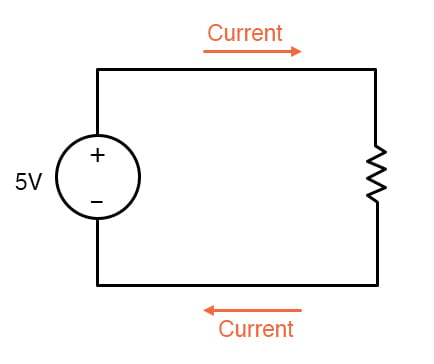If you are accustomed to thinking about electricity as the movement of electrons, which flow from lower voltage to higher voltage, you need to remember that in the context of circuit design and analysis, we assume that current is the movement of electric charge from higher voltage to lower voltage.

## Kirchhoff’s Current Law

This law, abbreviated KCL, states that the total current entering a node is equal to the total current exiting a node. This follows naturally from the idea that electric charge cannot disappear. KCL allows us to find unknown branch currents by way of known branch currents. In the example below, if we measure I1 and I2, we can calculate I3, because I1 = I2 + I3.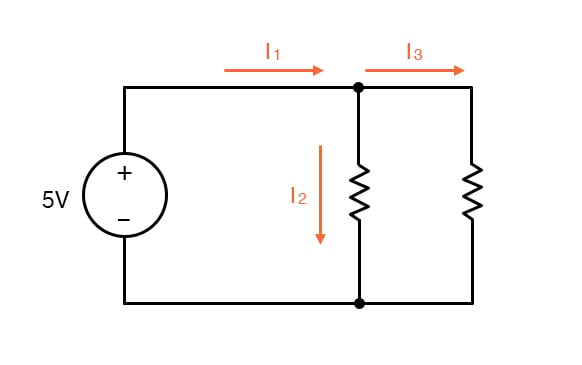## Kirchhoff’s Voltage Law

A typical circuit consists of a voltage source and various components that “claim” part of this supplied voltage as a result of their electrical behavior. The change in voltage between one terminal of a component and another terminal of the same component is called a voltage drop

Kirchhoff’s Voltage Law, abbreviated KVL, tells us that the sum of the voltage drops in a closed circuit is equal to the voltage supplied by the source. In other words, all of the voltage generated by the source must be accounted for somewhere in the circuit. In the example below, we have used a voltmeter to find the voltage across R1. Since VR1 = 2 V and VSUPPLY = 5 V, we know from KVL that VR2 = 3 V.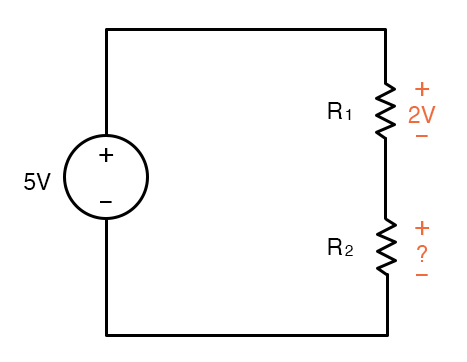## Ohm’s Law

We have already learned about voltage sources and resistance, and we know that a voltage source will generate current flow when it is connected to a circuit consisting of one or more resistive components. In order to determine the exact amount of current, we need Ohm’s Law, which is an indispensable piece of information for anyone who works with electronic circuits. It states that the current through a resistive component is equal to the voltage across the component divided by the resistance.

$I=\frac {V}{R}$

We can rearrange this equation as follows:

$V =IR$

In this form, Ohm’s Law tells us that the voltage across a resistive component is equal to the current flowing through the component multiplied by the resistance. The diagram below shows how Ohm’s Law can be used to determine, first, the total current in the circuit, and second, the voltage dropped across each resistor.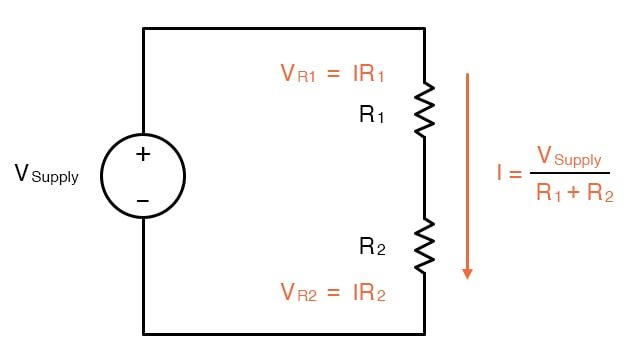## Power Dissipation

Neither voltage nor current is a direct indication of the way in which a circuit is using energy. However, energetic calculations are very important because energy, not voltage or current, is the quantity that corresponds to a system’s ability to perform useful work.

The energetic characteristics of an electric circuit or component are analyzed by means of power, which tells us the rate at which energy is consumed or transferred. Electric power (in watts) is the product of voltage (in volts) and current (in amperes):

$P=IV$

By combining this equation with Ohm’s Law, we can create alternative expressions that emphasize the relationship between power and voltage or between power and current:

$P=\frac {V^2}{R}$

$P=I^2R$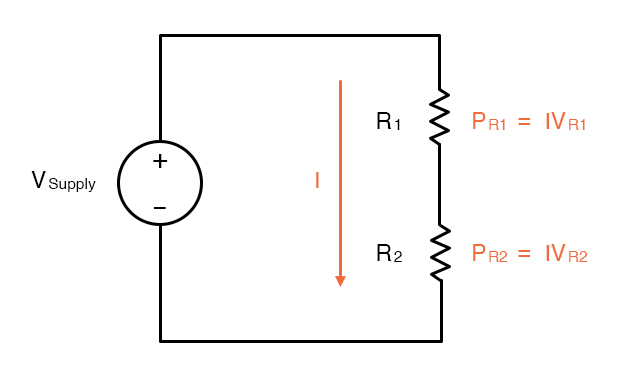Resistive materials convert electrical energy into heat. In most cases this heat is not desired. Consequently, the power associated with a resistive material is “dissipated” into the environment, and we often refer to electrical power as power dissipation.

## Recap

• Electric circuits are energetic systems in which we analyze currents, voltages, and power dissipation.
• For the purposes of circuit analysis, electric current flows from higher voltage to lower voltage.
• The behavior of electric current at a node and the behavior of voltages in a closed circuit are described, respectively, by Kirchhoff’s Current Law and Kirchhoff’s Voltage Law.
• Ohm’s Law states that the current flowing through a resistive component is equal to the voltage across the component divided by its resistance.
• Power dissipation is equal to voltage multiplied by current.